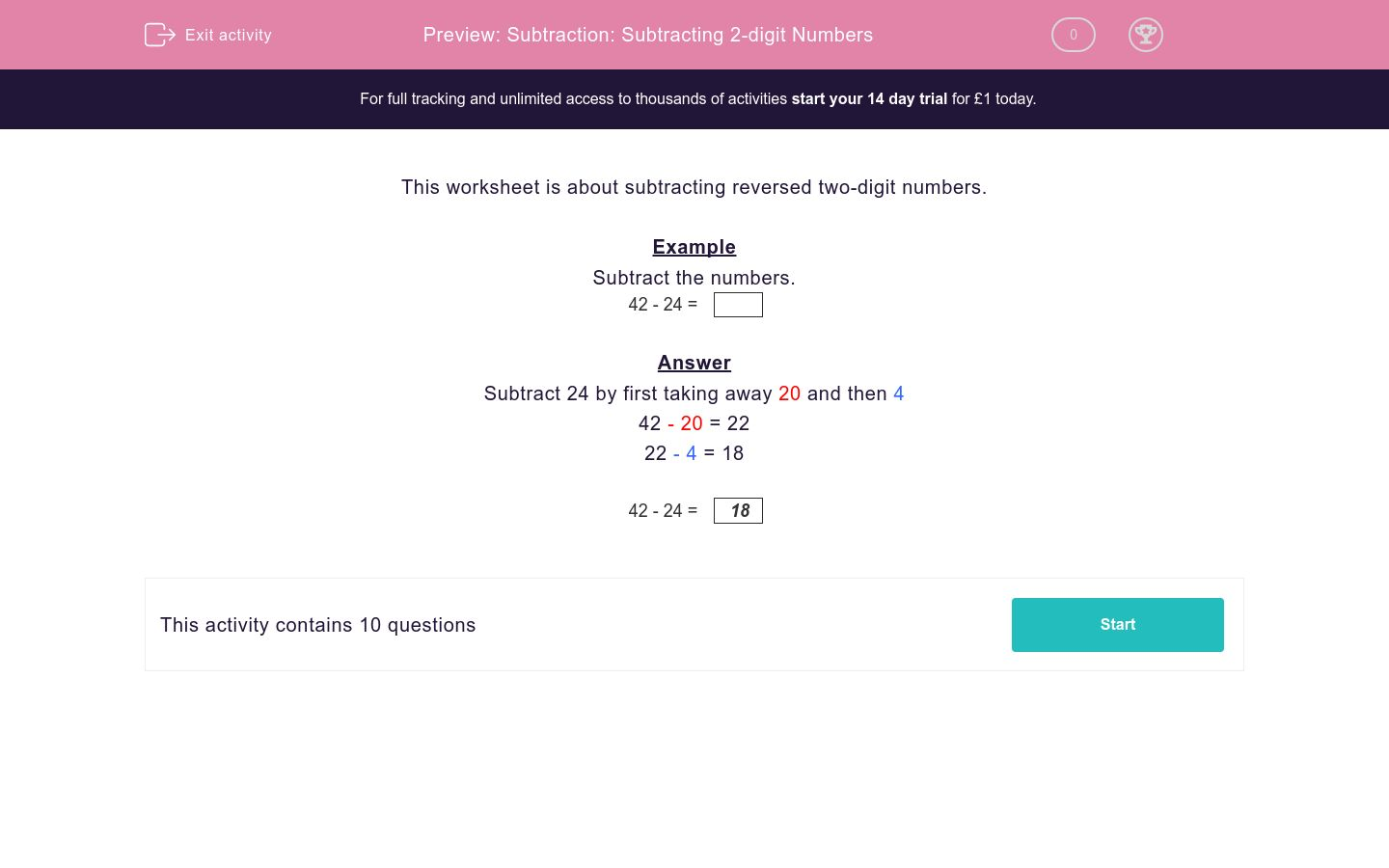# Subtraction: Subtracting 2-digit Numbers

In this worksheet, students subtract reversed two-digit numbers and noticing a pattern at the end.Key stage:  KS 2

Curriculum topic:   Maths and Numerical Reasoning

Curriculum subtopic:   Mixed Problems

Difficulty level:### QUESTION 1 of 10

This worksheet is about subtracting reversed two-digit numbers.

Example

Subtract the numbers.

42 - 24 =

Subtract 24 by first taking away 20 and then 4

42 - 20 = 22

22 - 4 = 18

42 - 24 =
 18

---- OR ----